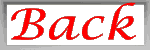# Probability Notes Bi

 ProbabilityProbability Facts:

• Probability is the likelihood that an event will occur from random chance
• Probabilities are always between 0 and 1
• Probabilities can be written as fractions, decimals or percentages
• All possible events together must have a probability of 1
• The probability that an event will not occur is 0
• To find the probability of a particular event, we use this formula:

 Number of successful events (where a successful event is the result that you are looking ) Probability of an event = ——————————————— Total number of events

• Whenever a coin is flipped, there is a 50% chance of getting heads & a 50% chance of getting tails.
• The chance of inheriting one of two alleles from a parent is also 50%

Applying Probability to Mendel’s Peas:

• During the late 1850’s, Gregor Mendel used the general rules of probability to explain the basic principles of heredity by breeding green peas in planned experiments
• Different versions of genes are called alleles
• For most genes, two alleles exist
• If an organism has two different alleles for the same gene, the dominant allele will be expressed in the phenotype of the organism, while the recessive allele will not
• Each parent will pass on one of its two alleles

Probability Laws:

• The Law of Multiplication or Product Rule states that the chance of two or more independent events occurring together is the product of the probability of the events occurring separately
Example: The chance of inheriting a specific allele from one parent & another allele from the other parent is 1/2  x  1/2 or 1/4
• The following table shows the probability of the offspring genotypes whenever two heterozygous parents are crossed:

 Parents     Rr  x  Rr Offpring  RR 1/2  x  1/2  = 1/4 Offpring  Rr 1/2  x  1/2  = 1/4 Offpring   r r 1/2  x  1/2  = 1/4

Complete the following table for crossing 2 Homozygous parents:

 Parents     RR  x  rr Offpring  Rr

Example 1
Imagine we are rolling a fair dice. There are six equally likely outcomes: 1, 2, 3, 4, 5 and 6.

1. What is the probability of getting a five?
In this case there is only one successful outcome or event, 5.

 Number of successful events Probability of a five = ——————————————— Total number of events 1 Probability of a five = ———- 6

1. What is the probability of getting an even number?
In this case there are 3 successful outcomes, which are 2, 4 and 6.

 Number of successful outcomes Probability of an even number = ———————————— Total number of outcomes 3 Probability of an even number = ———- 6 1 Probability of an even number = ———- 2

Therefore the probability of getting an even number when rolling a dice is 50% or 1/2.

• Now apply these numbers to the dihybrid cross RrYy  x  RrYy Probability of being R_ Y_ is 3/4 x 3/4 = 9/16  Probability of being R_ y_ is 3/4 x 1/4 = 3/16  Probability of being r_Y_ is 1/4 x 3/4 = 3/16 Probability of being r ryy is 1/4 x 1/4 = 1/16 This matches our 9:3:3:1 phenotypic ratio.

Example Problem:

Work a trihybrid cross of heterozygous parents   —    RrYyGg   x   RrYyGg

1. What is the probability of having a dominant gene for all three traits?
2. What is the probability of being recessive for all three traits?
3. What is the probability of being dominant for 2 and recessive for one?
4. What is the probability of being dominant for 1 and recessive for two?